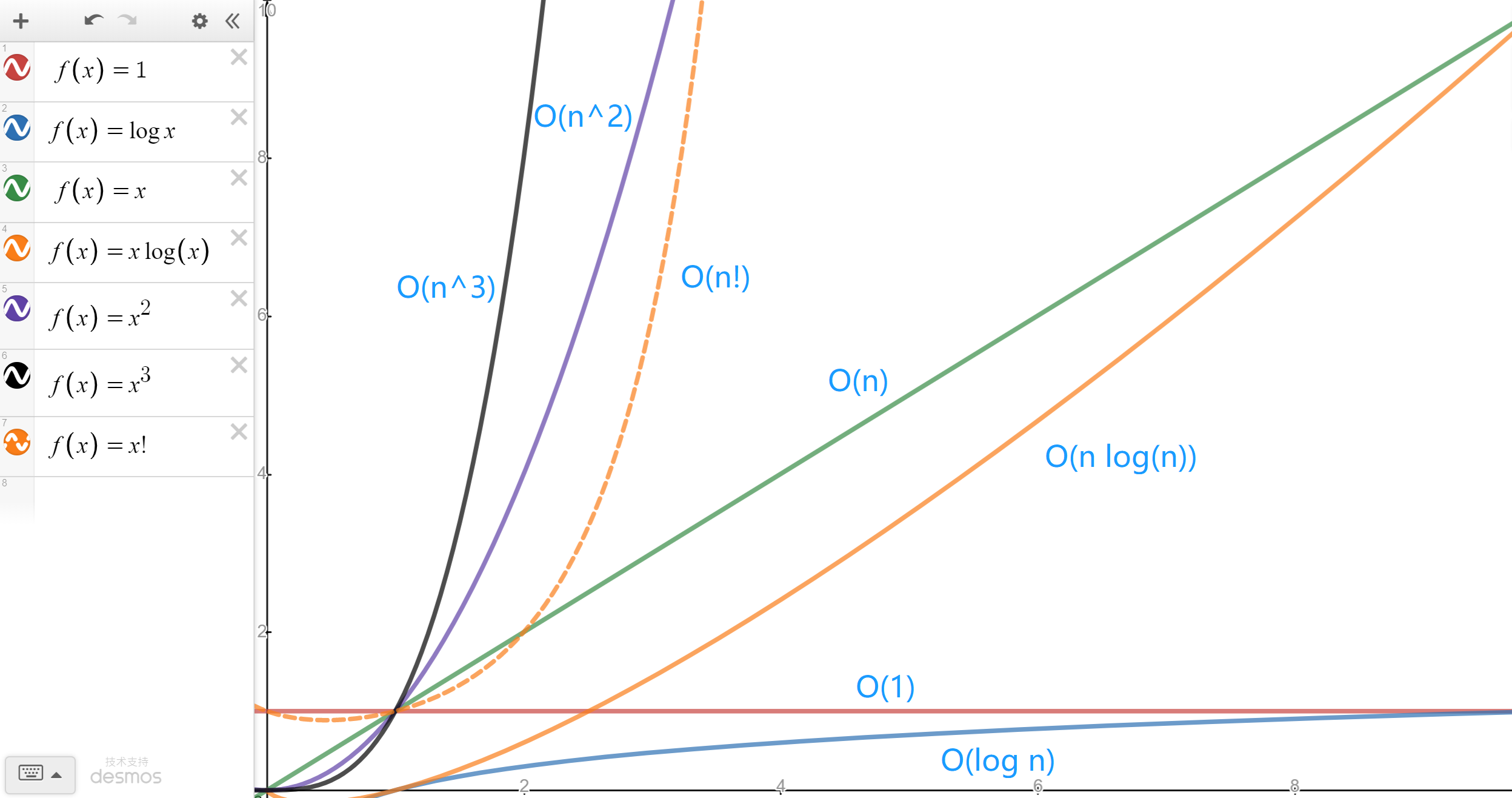# 算法简介

• 输入
• 输出
• 有穷性
• 确定性
• 可行性
1. 输入 & 输出

1. 有穷性

1. 确定性

1. 可行性

## # 算法的设计要求

1. 正确性

• 算法程序无语法错误。
• 算法程序对于合法的输入能得到满足要求的输出。
• 算法程序对于非法的输入能得到满足规格说明的输出。
• 算法程序对于的特殊的测试数据也有满足要求的输出。
1. 可读性

1. 健壮性

1. 高时间效率

1. 低存储量

## # 算法效率衡量方法

### # 时间复杂度

#### # 推导时间复杂度的原则

1. 若运行时间是常数量级，则用常数 1 表示
2. 只保留时间函数中最高阶项，如 $O(n^2 + 4n)$，保留最高阶项后，成为 $O(n^2)$
3. 若最高阶项存在，则省去最高阶项前的系数，如 $O(4n^2)$，省去最高阶项的系数后，成为 $O(n^2)$

#### # 分析时间复杂度的方法

1. 只关注循环执行次数最多的一行代码；
2. 加法原则：总复杂度等于量度最大的那段代码的复杂度；
3. 乘法原则：嵌套代码的复杂度等于嵌套内外代码复杂度的乘积

#### # 常见的时间复杂度曲线#### # 常见时间复杂度

##### #$O(1)$

void sayHello(String name){
System.out.prinln("Hello, " + String);
System.out.prinln("欢迎关注我的公众号：【村雨遥】");
}

##### #$O(log n)$

int binarySearch(int[] arr, int target){
int left = 0;
int right = arr.length - 1;
while(left <= right){
int middle = left + (left - right) / 2;
if(arr[middle] == target){
return middle;
}else if(arr[middle] > target){
right = middle - 1;
}else {
left = middle + 1;
}
}

return -1;
}

##### #$O(n)$

int sum(int[] arr){
int total = 0;

for(int i = 0; i < arr.length; i++){
total += arr[i];
}

}

##### #$O(n log n)$

void hello (int n){
for( int i = 1 ; i < n ; i++){
int m = 1;
while( m < n ){
m *= 2;
}
}
}

##### #$O(n^2)$

void selectionSort(int[] arr, int n){
for(int i = 0; i < n; i++){
int min = i;
for(int j = i + 1; j < n; j++){
if(arr[j] < arr[min]){
int tmp = arr[i];
arr[i] = arr[j];
arr[j] = tmp;
}
}
}
}

##### #$O(n^3)$

$O(n^2)$，类似，将时间复杂度为 $O(n^2)$ 的代码嵌套循环一次，此时复杂度就变成了 $O(n^3)$，表现出来就是三重循环嵌套的形式。

void demo(int n){
for(int i = 0; i < n; i++){
for(int j = 0; j < n; j++){
for(int k = 0; k < n; k++){
System.out.print("Hello, World");
}
System.out.print("------");
}
System.out.print("******");
}
}


### # 空间复杂度

#### # 常用空间复杂度

##### #$O(1)$

int num1 = 1;
int num2 = 2;
int total = num1 + num2;

##### #$O(n)$

int[] arr = new int[n];

##### #$O(n^2)$

int[][] arr = new int[n][n];